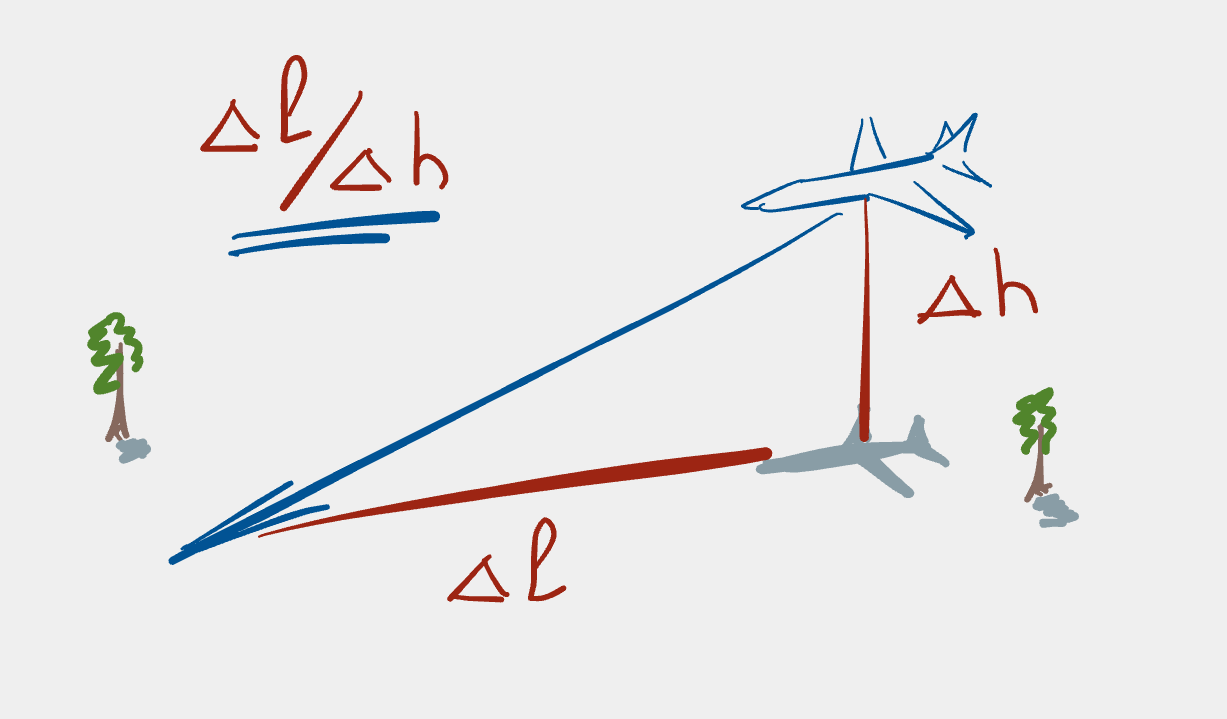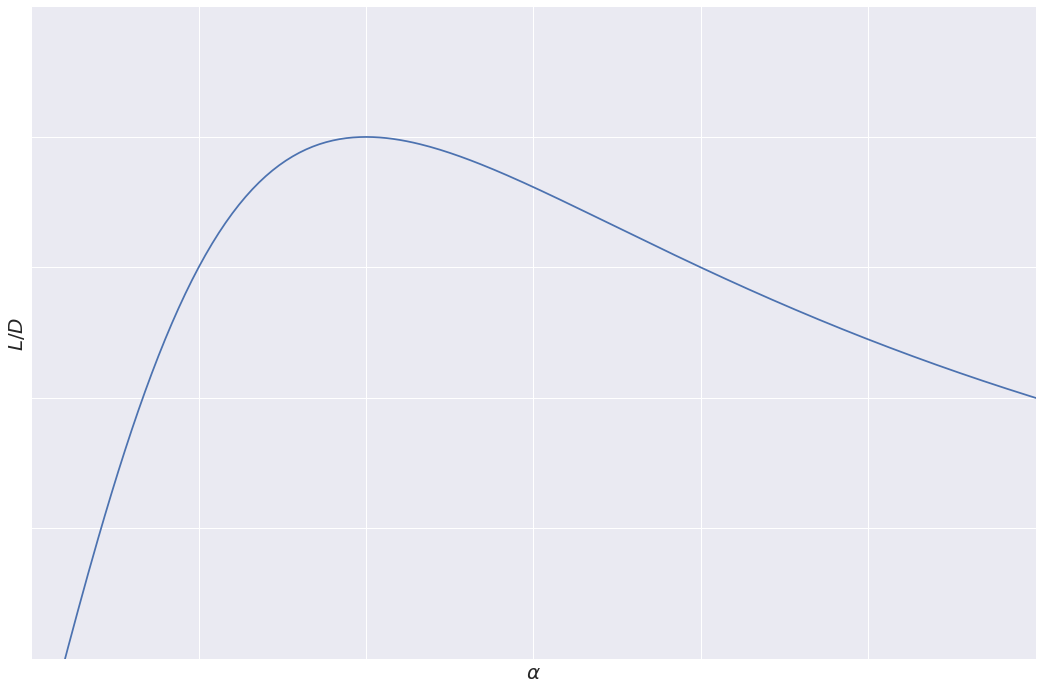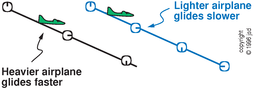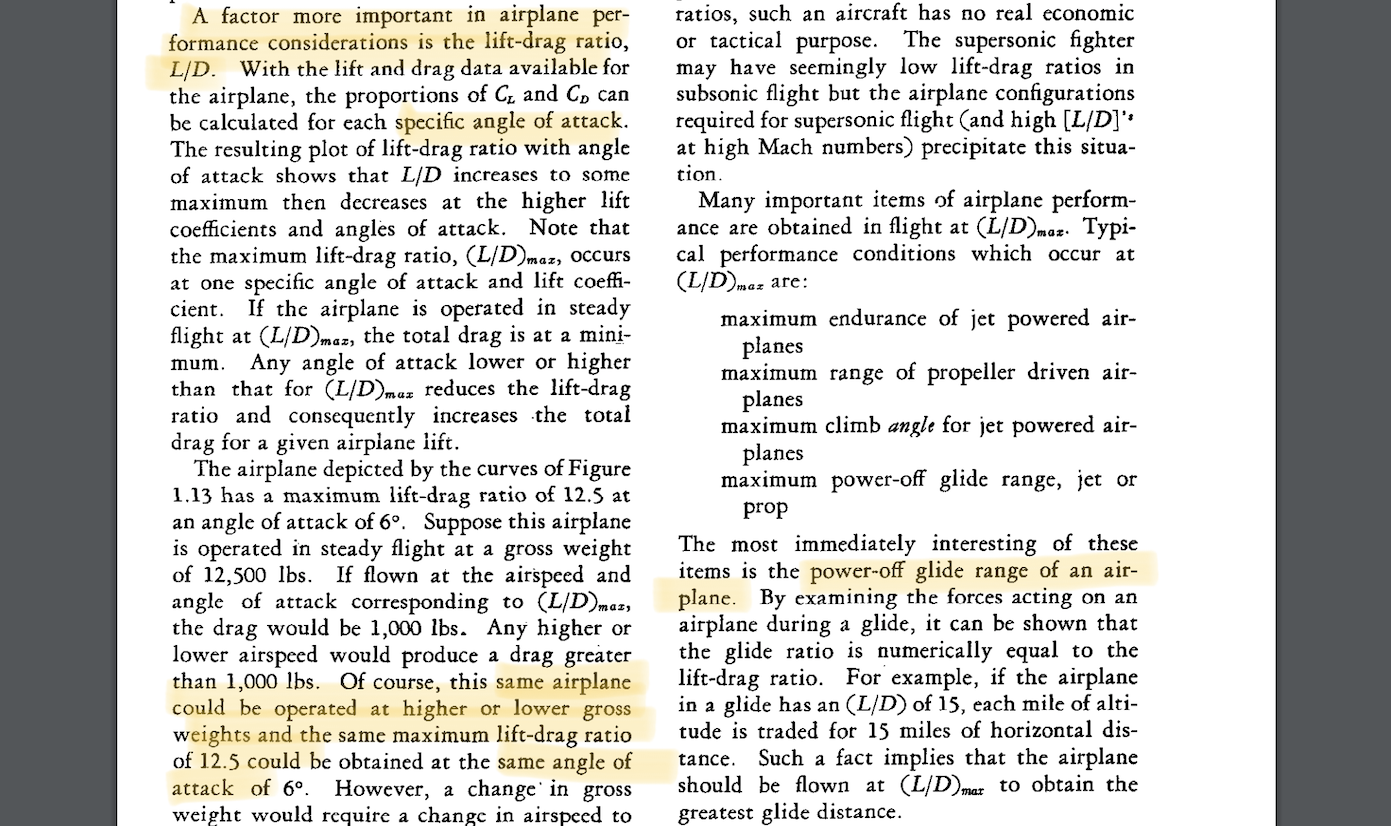# Glide ratio, lift-to-drag and their (in)dependence on aircraft weight

25 Jan 2021 • 9 min read
Tags:

TL;DR
Glide ratio is the distance a glider (aircraft without an engine or the engine switched off) covers for each unit of altitude lost while descending – e.g. 14:1 means 14 km of distance per 1 km of altitude. Best glide ratio is the most optimal - the highest - one. In this post, we will try to give a simple yet correct explanation of why this best glide ratio a) equals lift-to-drag ratio and b) does not depend on the weight of the aircraft.

Telegram-channel (in Russian): Crosswind LandingParadox: the distance a glider covers per each unit of altitude does not depend on how heavy the glider is.

## Motivation

Does the best glide ratio depend on the weight of the aircraft? When one searches the answer on the Internet, one gets something like that (italic mine):

Variations in aircraft weight do not affect the glide angle provided that the correct airspeed is flown. Since it is the lift over drag (L/D) ratio that determines the gliding range, weight will not affect it. Glide ratio is based only on the relationship of the aerodynamic forces acting on the aircraft. The only effect weight has is to vary the time the aircraft will glide for. The heavier the aircraft is, the higher the airspeed must be to obtain the same glide ratio.
— From www.skybrary.aero

While this is technically enough to pass your PPL (or any other xxxPL exam) theory exam, it – in my personal opinion – does not give you a solid foundation of the mechanics of this fact.

## Let’s get hands dirty!

So, let’s proceed to the derivation.

### Notation

Notation Meaning
$\alpha$ angle of attack (AoA)
$E$, $E_\text{kin}$, $E_\text{pot}$ total, kinetic and potential energy of the system
$\Delta h$, $\Delta \ell$ altitude and horizontal distance travelled (absolute values)
$D$ (incl. $D^p$, $D^i$), $L$ drag (incl. parasite and induced) and lift forces (absolute values)
$C_D$ (incl. $C_D^p$, $C_D^i$), $C_L$ drag (incl. parasite and induced) and lift coefficients
$V$ airspeed
$A_D$ work of the drag force (absolute value)

### Best glide ratio equals lift-to-drag one

First, note that gliding is engineless process, so there’s no energy input into our system and the only energy change is caused by the work of the drag force: $$\Delta E = A_D.$$

Second, we assume stable, non-accelerated gliding, so kinetic energy is constant: $$E_\mathrm{kin} = \mathrm{const},$$ and more importantly, we can operate with the speed related forces as constant ones. Among others, this implies that the drag force $D$ is constant and its drag force multiplied by the horizontal distance travelled (see $(\ddagger)$ below).

Taking into account the facts above, write down the energy preservation:

\begin{align} \Delta E &= A_D \quad &&\Longleftrightarrow \vphantom{\stackrel{1}{\Longleftrightarrow}} \notag \\ \Delta E_\text{kin} + \Delta E_\text{pot} &= A_D &&\Longleftrightarrow \vphantom{\stackrel{1 }{\Longleftrightarrow}} \notag \\ \Delta E_\text{pot} &= A_D &&\Longleftrightarrow \vphantom{\stackrel{1}{\Longleftrightarrow}} \notag \\ - m g \Delta h &= A_D &&\stackrel{(\dagger)}{\Longleftrightarrow} \vphantom{\stackrel{1}{\Longleftrightarrow}} \notag \\ - m g \Delta h &= - D \Delta \ell &&\stackrel{(\ddagger)}{\Longleftrightarrow} \vphantom{\stackrel{1}{\Longleftrightarrow}} \notag \\ - L \Delta h &= - D \Delta \ell &&\Longleftrightarrow \vphantom{\stackrel{1}{\Longleftrightarrow}} \notag \\ \Delta \ell / \Delta h &= L / D. \vphantom{\stackrel{1}{\Longleftrightarrow}} \label{ld} \end{align}

where transition $(\dagger)$ uses the fact that the work of drag force $A_D$ equals drag force $D$ multiplied by the horizontal distance travelled $\Delta \ell$, and transition $(\ddagger)$ uses a common assumption that lift force equals weight. Both assumptions are based on the fact that the trajectory is flat and the slanted vectors can be approximated by vertical/horizontal.

### Lift-to-drag ratio only depends on the angle of attack

#### Lift and drag in terms of angle of attack

Let’s write $L$ and $D$ in terms of AoA $\alpha$ and use $\eqref{ld}$. Important here is to not overcomplicate things and make useful approximations. For example, we are not going to consider the stalling regime (high $\alpha$).

Lift force is proportional to the lift coefficient $C_L$ which is linear in the AoA:

\begin{equation}\label{eq:lift} L = C_L V^2 \cdot \underbrace{\mathrm{const}}_{\substack{\text{env and acft} \\ \text{properties}}} \approx \alpha V^2 \cdot C_1. \end{equation}

Drag force, in turn, depends on the drag coefficient $C_D$ which we need to quickly derive. It consists of1:

• the parasitic drag, which is the resistance due to the shape plus some friction, and whose coefficient $C_D^p$ is approximately constant unless we fly some aerobatics at high AoA:

\begin{equation}\label{eq:par} D^p = C_D^p V^2 \cdot \underbrace{ \mathrm{const}}_{\substack{\text{env and acft} \\ \text{properties}}} \approx V^2 \cdot C_2. \end{equation}
• induced drag, which is essentially due to the wing tilted back as AoA grows, and so grows the backward component of the lift force2, and whose coefficient is growing approximately quadratically in AoA3:

\begin{equation}\label{eq:ind} D^i = C_D^i V^2 \cdot \underbrace{ \mathrm{const}}_{\substack{\text{env and acft} \\ \text{properties}}} \approx \alpha^2 V^2 \cdot C_3. \end{equation}

So, putting \eqref{eq:par} and \eqref{eq:ind} together, the total drag would be something like

\begin{equation}\label{eq:total} D = D^p + D^i \approx (C_4 + \alpha^2) V^2 \cdot C_5, \end{equation}

where we again moved unimportant parts into constants to unclutter things.

#### Lift-to-drag ratio in terms of angle of attack

Finally, let’s compute $L/D$ ratio using what we’ve just derived in lift \eqref{eq:lift} and total drag \eqref{eq:total} equations:

\begin{equation}\label{eq:ld-final} L/D \approx \frac{\alpha V^2 \cdot C_1}{(C_4 + \alpha^2) V^2 \cdot C_5} = C_6 \cdot \frac{\alpha}{(C_4 + \alpha^2)} \end{equation}

It turns out – the $L/D$ ratio is just a function of $\alpha$ and certain aircraft/environment properties (those latter account for various constants)!4 As expected, it has a maximum:We intentionally do not place units here, because all the calculations hold up to constants and hence precise numbers do not make sense anyway.5

Same can be seen mathematically: assuming both constants in \eqref{eq:ld-final} are unit, the derivative of $L/D$ equals

$$\bigl(L/D\bigr)'_{\alpha} = \frac{1 + \alpha^2 - 2 \alpha^2}{(1 + \alpha^2)^2} = \frac{1 - \alpha^2}{(1 + \alpha^2)^2},$$

and equals zero at an unscaled value $\alpha = 1$ (again, no precise meaning of this particular number because of constants all around), and with one more step one can check that this is maximum.

This means exactly that the weight of the aircraft does not influence lift-to-drag and glide ratios, the latter reaching the best optimum at the same angle of attack.

## Other resources worth checking

First, I can’t help citing a beautiful online book “See How It Flies” written by a physicist, one of the early neural network researchers (!) and a passionate flight instructor John S. Denker:

The lightly-loaded gliding airplane will have the same angle of descent, the same direction of flight, and the same total gliding distance, as indicated in figure 2.18. The only difference is that it will have a slower descent rate and a slower forward speed; this is indicated in the figure by stopwatches that show how long it takes the plane to reach a particular point.© John S. Denker, source

— From http://www.av8n.com

Second, another citation of a wonderful source, old but good “Aerodynamics for naval aviators” from 1965:

Any angle of attack lower or higher than that for $(L/D)_\text{max}$ reduces the lift-drag ratio and consequently increases -the total drag for a given airplane lift.
<…> However, a change’ in gross weight would require a change in airspeed to support the new weight at the same lift coefficient and angle of attack.Screenshot of “Aerodynamics for naval aviators”

— From p. 28 of “Aerodynamics for naval aviators”

## Conclusion

We’ve shown that

• Glide ratio is equal to lift-to-drag one;
• Lift-to-drag ratio only depends on angle of attack (and aircraft/wing shape or environment properties – which are fixed);
• Hence, the best glide ratio is achieved only at the given optimal angle of attack, independent of weight.

## Acknowledgements

Many thanks to Dr. Yulia Krasnikova (@little_aviator) who took time to review this post and provided useful suggestions!

1. Please note that I’ve made some simplifications here, not necessarily following the word and letter of textbooks. There are a lot more components to those equations and a lot more different regimes in which those equations transform. But at this stage, the aim is to understand the general, yet correct, intuition of how those equations relate to each other, and this is fullfilled. ↩︎

2. There exists quite a variety of views on the correct way to explain the cause of induced drag. Some say it is due to wing vortices, some say due to downwash behind the wind. I feel those are both manifestation of the same physical effect which Zhukovsky called circulation, and this duality allows different yet correct views. ↩︎

3. In the spirit of dropping unnecessary complexity, I prefer to think of the cause for this as follows: there is a lift force, which is proportional to $\alpha$, - tilted back by a small angle which turns out to be proportional to $\alpha$, – giving $\alpha \cdot \sin \alpha \approx \alpha^2$ coefficient. See a Wikipedia illustration. ↩︎

4. To recap – among those such things as air density, wing aspect ration, wing span, aircraft shape cross-section area, and many more. ↩︎

5. Another important thing to notice is the behavior of this curve towards higher AoA: the curve in reality must go steeper down because of the stall regime, but it is not depicted here precisely for the reason of simplification and leaving stall out of consideration. ↩︎